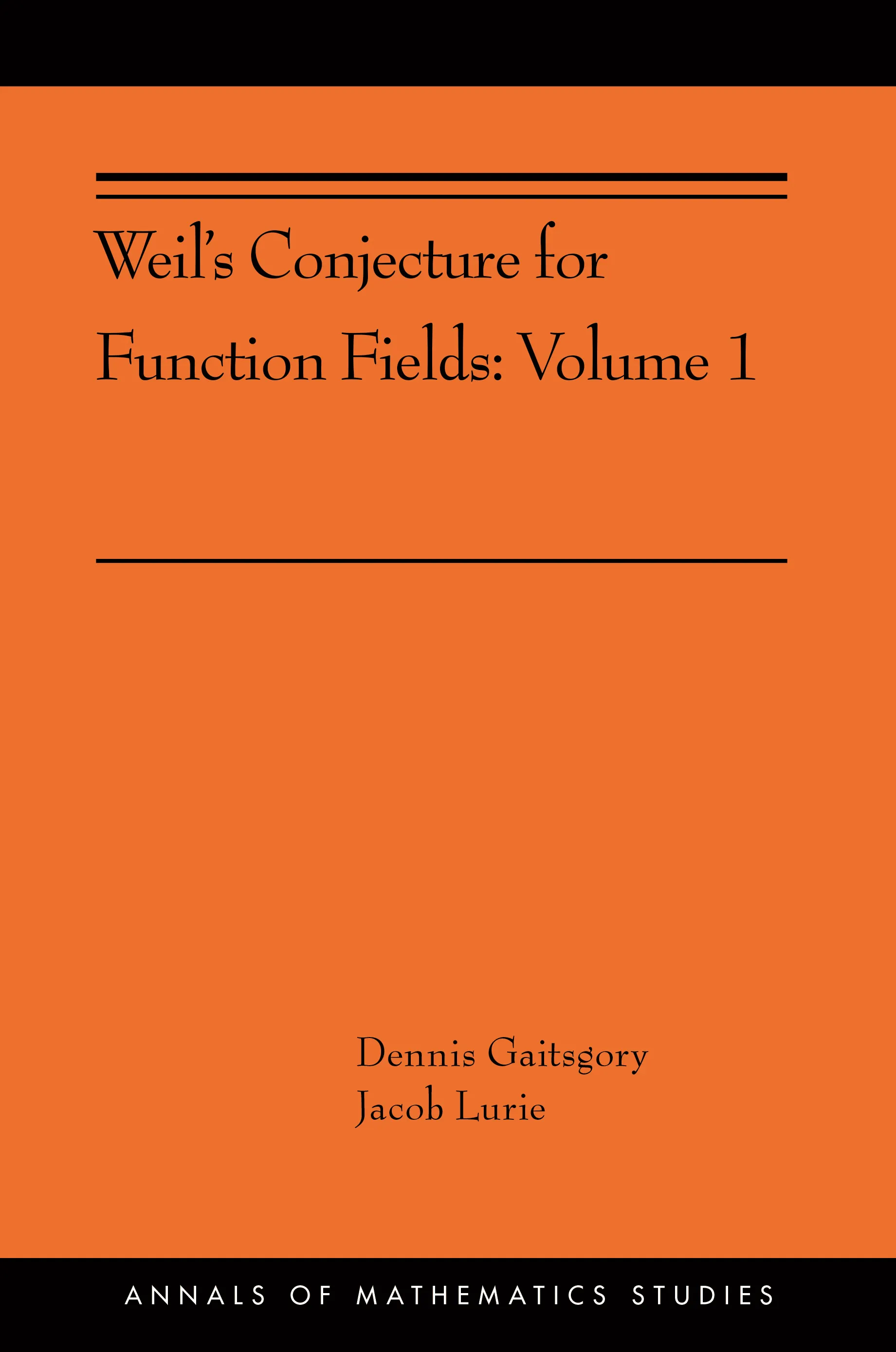#### Weil's Conjecture for Function Fields

Dennis Gaitsgory

##### ebooks

Many of our ebooks are available for purchase from these online vendors:

Many of our ebooks are available through library electronic resources including these platforms:

# Weil's Conjecture for Function Fields: Volume I (AMS-199)## Hardcover

Price:
\$165.00 / £136.00
ISBN:
Published:
Feb 19, 2019
2019
Pages:
320
Size:
6.12 x 9.25 in.

A central concern of number theory is the study of local-to-global principles, which describe the behavior of a global field K in terms of the behavior of various completions of K. This book looks at a specific example of a local-to-global principle: Weil’s conjecture on the Tamagawa number of a semisimple algebraic group G over K. In the case where K is the function field of an algebraic curve X, this conjecture counts the number of G-bundles on X (global information) in terms of the reduction of G at the points of X (local information). The goal of this book is to give a conceptual proof of Weil’s conjecture, based on the geometry of the moduli stack of G-bundles. Inspired by ideas from algebraic topology, it introduces a theory of factorization homology in the setting ℓ-adic sheaves. Using this theory, Dennis Gaitsgory and Jacob Lurie articulate a different local-to-global principle: a product formula that expresses the cohomology of the moduli stack of G-bundles (a global object) as a tensor product of local factors.

Using a version of the Grothendieck-Lefschetz trace formula, Gaitsgory and Lurie show that this product formula implies Weil’s conjecture. The proof of the product formula will appear in a sequel volume.